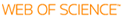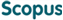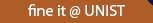### BROWSE

#### Related ResearcherKang, Sang Hoon
Robotics and Rehab. Engineering Lab (R2EL)
Research Interests
• Rehabilitation Robotics & Mechatronic Tools, Biomechanics for Rehabilitation, Human Limb Impedance Estimation, Assistive and Healthcare robotics, Robust Motion/Force Control

Efficient Acceleration-Level Formula of Bias Acceleration Satisfying Time Precedence for Operational Space Formulation

Cited 0 times inCited 0 times inTitle
Efficient Acceleration-Level Formula of Bias Acceleration Satisfying Time Precedence for Operational Space Formulation
Author
Issue Date
2022-06
Publisher
Institute of Electrical and Electronics Engineers Inc.
Citation
IEEE ACCESS, v.10, pp.65533 - 65547
Abstract
Operational space formulation has been used for robots to perform multi-tasks by taking advantage of dynamic consistency. Bias acceleration, which is an acceleration realized right after applying the control torque to a robot, compensates for the acceleration due to the higher priority tasks, thereby achieving the goal of the tasks with lower priority. Since the operational space formulation requires many computations during real-time control, there is a strong need to reduce the number of computations. We proposed an efficient acceleration-level bias acceleration formula while showing that the original bias acceleration formula could take 30-50% of the total computation for the operational space formulation. Moreover, we clarified the underlying time precedence of bias acceleration, which was implicitly provided in the original formula but was never clearly explained. Further, we have proved that the inefficiency of the original formula is due to the use of unnecessary full robot dynamics, the use of which is almost entirely eliminated in the proposed formula. Through extensive simulations and experiments, we have verified that the proposed formula needs 2-4% computations of the original, is independent of the Coriolis/centrifugal torque and the gravity torque, and is equivalent to the original formula. We expect that, with a deeper understanding of the bias acceleration, the proposed bias acceleration formula could promote the use of high degrees-of-freedom robots.
URI
https://scholarworks.unist.ac.kr/handle/201301/59042
DOI
10.1109/ACCESS.2022.3183609
ISSN
2169-3536
Appears in Collections:
MEN_Journal Papers
Files in This Item:can give you direct access to the published full text of this article. (UNISTARs only)